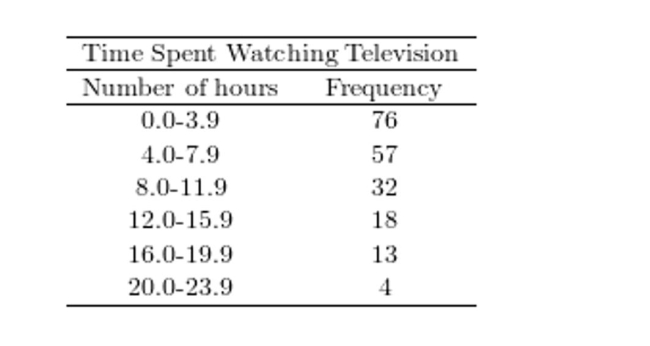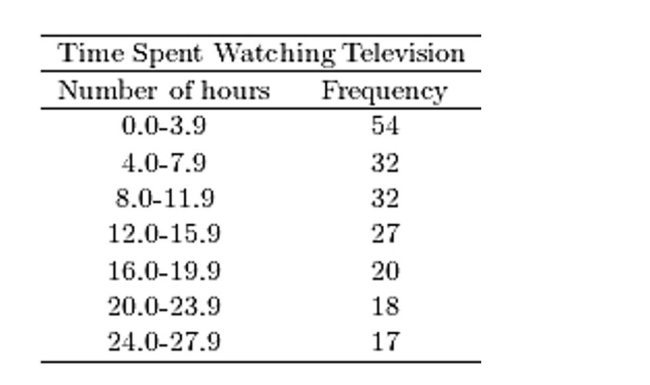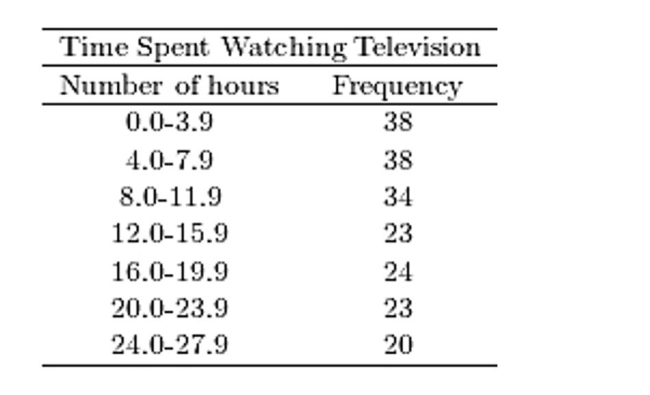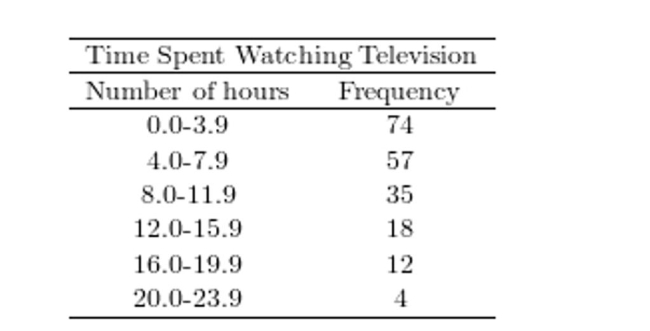Try out our new practice tests completely free!# Quiz 2: Graphical Summaries of DataWhich of the following presents the most honest graphical representation of the ratio "3 to 4"?
(Multiple Choice)Which of the following presents the most honest graphical representation of the ratio "5 to 2"?
(Multiple Choice)Construct a dotplot for the following data. 3.99 4.02 3.97 3.94 3.94 3.92 3.91 3.91 3.91 4.04 3.98 3.94 3.96 3.97 3.94 3.99 3.93 3.90 3.97 3.99
(Multiple Choice)Thirty households were surveyed for the number of televisions in each home. Following are the results. 1 0 0 2 1 2 4 2 1 1 0 0 0 0 1 5 0 2 0 0 0 0 2 1 0 0 5 5 3 0 Construct a frequency histogram.
(Multiple Choice)Construct a dotplot for the following data. 15 15 15 14 13 13 14 15 13 15 14 15 14 13 17 15 14 12 16 15
(Multiple Choice)Following are the numbers of Dean's List students in a random sample of 20 university courses. Construct a dotplot for these data. 9 2 0 0 4 2 0 0 4 0 4 2 0 0 5 6 1 2 0 4
(Multiple Choice)A sample of 200 high school students were asked how many hours per week they spend watching television.The following frequency distribution presents the results.Construct a relative frequency ogive for the frequency distribution.
(Multiple Choice)Construct a stem-and-leaf plot for the following data. 22 38 51 12 57 33 67 20 31 29 19 48 19 31 29 53 54 21 22 55
(Multiple Choice)Thirty households were surveyed for the number of televisions in each home. Following are the results. 4 0 4 3 0 0 4 1 0 4 0 1 1 0 1 1 5 2 5 1 3 0 3 0 1 0 3 2 3 0 Construct a relative frequency histogram.
(Multiple Choice)A sample of 200 high school students were asked how many hours per week they spend watching television.The following frequency distribution presents the results.Construct a frequency polygon for the frequency distribution.
(Multiple Choice)Construct a stem-and-leaf plot for the following data, in which the leaf represents the tenths place. 8.9 6.7 4.3 9.9 9.3 10.6 9.5 7.8 3.0 5.3 8.1 10.6 6.1 9.2 10.4 9.7 9.8 10.6 6.8 3.0 7.6 9.3 3.9 6.2
(Multiple Choice)A sample of 200 high school students were asked how many hours per week they spend watching television.The following frequency distribution presents the results.Construct a frequency ogive for the frequency distribution.
(Multiple Choice)Which of the following presents the most honest graphical representation of the ratio "3 to 2"?
(Multiple Choice)A sample of 200 high school students were asked how many hours per week they spend watching television.The following frequency distribution presents the results.Construct a relative frequency polygon for the frequency distribution.
(Multiple Choice)## Filters

• Essay(0)
• Multiple Choice(0)